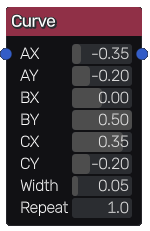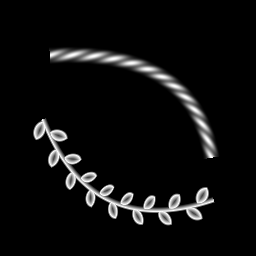# Curve node¶

The Curve node outputs a simple RGBA image showing a bezier curve defined by 3 control points.## Inputs¶

The Curve node accepts an optional image that is mapped along the curve.

## Outputs¶

The Curve node generates an RGBA image showing the bezier curve.

## Parameters¶

The Curve node has the following parameters:

• the coordinates of the control points

• the width of the curve to be drawn

• the number of repetitions of the input pattern along the curve

## Example images¶